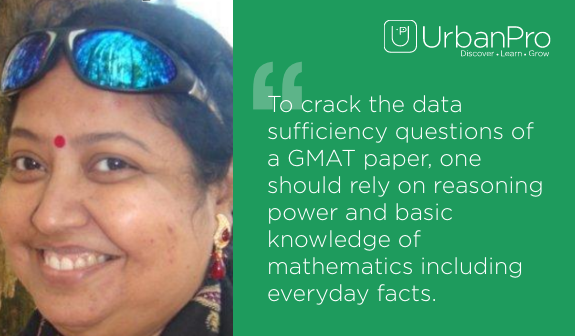Find the best tutors and institutes for GMAT Coaching

Find Best GMAT Coaching classes

No matching category found.

No matching Locality found.

Outside India?

Search for topics

# Data Sufficiency of GMAT exam explained by Anuradha DattaAnuradha Datta, a certified tutor from the British Council is also a verbal trainer for GMAT exam. Today, she discusses the Data Sufficiency portion of a GMAT paper for easy understanding of students and applicants.Tips, suggestions and examples make this interview a good read before you start solving or practicing Data Sufficiency related maths problems.

1)  Where does the data sufficiency section come in a typical GMAT question paper?
The data sufficiency maths problems comes in the quantitative section of a GMAT paper. Its right after the problem solving questions.

2)  How many data sufficiency questions are there to solve during GMAT exam?
In a GMAT exam, each candidate has to answer approximately 15 data sufficiency maths problems.

3)  How to pick the right answer from the five choices in data sufficiency problems?
Each data sufficiency problem of GMAT exam consists of a question and two statements, labeled (1) and (2), that give data. As far as data sufficiency question is concerned, you don’t need to arrive at a numerical solution.
You just have to decide whether the data given in the statements are sufficient for answering the question. Using the data given in the statements plus your knowledge of mathematics, everyday facts and reasoning power, you must indicate whether the data given in the statements are sufficient for answering the questions.
You may note that in data sufficiency problems that ask for the value of a quantity, the data given in the statement are sufficient only when it is possible to determine exactly one numerical value for the quantity.

4)  Which math chapters should an examinee practice to score maximum in the data sufficiency section of GMAT paper?
To crack the data sufficiency questions of a GMAT paper, one should rely on reasoning power and basic knowledge of mathematics including everyday facts.

5)  Is logical reasoning also required to solve data sufficiency problems during GMAT exam?
Yes

6)  Can you explain one data sufficiency math problem with vivid explanation?
Problem: Did candidate A receive more than half of the 30,000 votes cast in Corporation Election?

Directions: This data sufficiency problem consists of a question and two statements, labeled (1) and (2), in which certain data are given. You have to decide whether the data given in the statements are sufficient for answering the question. Using the data given in the statements, plus your knowledge of mathematics and everyday facts (such as the number of days in July or the meaning of the word counterclockwise), you must indicate whether:
A. Statement (1) ALONE is sufficient, but statement (2) alone is not sufficient to answer the question asked.
B. Statement (2) ALONE is sufficient, but statement (1) alone is not sufficient to answer the question asked.
C. BOTH statements (1) and (2) TOGETHER are sufficient to answer the question asked, but NEITHER statement ALONE is sufficient to answer the question asked.
E. Statements (1) and (2) TOGETHER are NOT sufficient to answer the question asked, and additional data specific to the problem are needed.

Solution: The answer to this question is either yes or no. So, ignore statement 2 and evaluate statement 1. Does statement alone answer the question? Many assume that there are only two candidates in the election (reason that if candidate B received 18,000 votes, then candidate A must have received 18,000 votes). However, there is no reason to believe that there were only two candidates. So, statement 1 is insufficient.
Write down BCE. Does statement 2 alone answer the question? Yes, it is pretty clear that candidate A received more than half of the votes (total votes cast is 30,000).

7)  Share three tips to deal with data sufficiency problems during GMAT exam.
The three tips for data sufficiency portion of GMAT exam are as follows:

a) Evaluate the statements one at a time before you think about combining them; read the question stem and only the first statement. Ignore the second statement. Explore all possibilities offered by each statement individually.
b) Be familiar with the answer choices. AD or BCE. These are always your options when you start eliminating. Memorize these.
c) Avoid paying too much attention to the statements and forget the rest of the question. Often important information is hidden in the prompt.

8)  How to solve figure based data sufficiency math problems during GMAT exam?

The following tips can be useful while solving figure-based Data Sufficiency problems:

a) A figure accompanying a data sufficiency question will conform to the information given in the question but will not necessarily conform to the additional information given in statements (1) and (2).
b) Lines shown as straight can be assumed to be straight and lines that appear jagged can also be assumed to be straight.
c) You may assume that the positions of points, angles, regions, etc. exist in the order shown and that angle measures are greater than zero.
d) All figures lie in a plane unless otherwise indicated. Figures may be altered as needed.

Connect with Anuradha Datta, a GMAT trainer for more queries and tips.

Read for more tips, patterns and suggestions to prepare for Integrated Reasoning and Quantitative section of GMAT exam.

How can we Improve it?

Please enter your question below and we will send it to our tutor communities to answer it *

Name *

Enter a valid name.

Email *

Enter a valid email.

Email or Mobile Number: *

Related Questions15/10/2018
5

Now ask question in any of the 1000+ Categories, and get Answers from Tutors and Trainers on UrbanPro.com